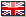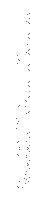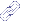★ APPLICATIONS ★ DIVERS ★ Giant Long Multiplication ★Giant Long Multiplication|The Amstrad User)Applications Divers

#### 123 - the multiplicand x 456 - the multiplier  738 - partial product 6150 - "       "49200 -"       "56088 - the product

First we take the units digits of the multiplier - that is a 6. Then each of the digits in the multiplicand is, in tufn, multiplied by the 6, taking into account the "carries". We thus start off with 6 by 3 = 18; that is 8 down and carry

1 (which isn't really one but a unit of ten which appears as a one in the tens column).

Next we move to the tens column of the multiplicand and have 6 by

2 = 12 with the 1 unit of ten carried over. So 12 +1 = 13, writedown the

3 and carry "1" (1 unit of 100 this time). It is the same process again, but with different numbers so we can use a loop.

Finally 6 by 1 = 6, plus 1 (unit of 100) carried over gives 7.We now have a partial product 738. The above process is repeated (which means we can use another loop) with the 5 in the multiplier (which is really a 50 not a 5) to get 6150, and yet again with the 4 (that is 400) to get 49200. The final stage is to add up the partial products to get the product (answer).

BUILDING UP THE PROGRAM

The multiplicand and muliplier are entered as strings, the multiplicand in line 140 as a\$ and the multiplier in 180 as b\$. Line 160 gets the number of characters (length) of a\$ and stores it as la, in line 190, lb docs the same for bS. Each string is then broken down into its separate digits, which, via the loops in line 270 for b\$ and 300 for a\$ are then stored in arrays a(j) and b(i).

In line 270, for the first time through the loop i=1 therefore b(i) is b(1). Now line 280 "reads" b(1) = VAL(MlD\$(b\$,1b,1)) If 1b = 4 then MID\$(b\$,4,1) means "what is the fourth digit in b\$, the 1 at the end meaning only one character is required. Suppose the fourth digit is a 9. We now have "b(1) is to be the value of a string containing just the cliaracter 9". b(1) is thus the number 9. The loop then increments to 2,3... up to its limit, picking out 1 digit at a time.

Note that b(1) is the first character counting from the right hand end [the same applies to a(i)]. b(3> is the third digit from the right, the one in the hundreds column.

For the example above 123 x 456, a(1)=3 and b(1)=6 so 6 x 3 is found by pp=a(1)*b(1) in line 320 (but ignore the +ca for the moment). So pp=18.

Now comes the fun of turning 18 into 8 and a 1 to carry over to the next stag2 (the tens column), this is done by using the WHILE-WEND loop structure (lines 340 to 370).

WHILE pp>9 (> means is greater than, < means is less than) which 18 is, then subtract 10 (line 350), and add 1 to the counter ca (line 360). As a result pp is now 8 and ca = 1, this now causes us to exit from this loop and continue. If pp is much greater than 9, such as 63, then the WHILE-WEND LOOP keeps operating, taking 10 off pp and adding 1 to ca on each pass. The final result in this example is pp=3 and ca=6 equivalent to "write down the 3 and carry 6" (ca for carry) So what happens to the current value of ca? On the next pass through the j loop ca is added to pp (line 320) and then immediately reset to zero (line 330) ready in case pp is greater than 9 (line 340) on the next pass through the loop. By the way, the i loop picks out one digit Irom the multiplier and the j loop then uses this with each digit in the multiplicand. Within the j loop each value of pp is printed as pr\$. The reason for this has to do with the formatting. PRINT pp will print (space)(digits). Since we don't want a space we have stripped it off using the RIGHTS command (line 390).

When the j loop has been completed we have to see if ca has a value higher than zero. This is the final carry and it has to be printed (line 430), we have used another WHILE-WEND routine to take care of this. Each time the i loop (line 270) is incremented we have to check whether we are multiplying by units, hundreds, thousands etc., so the appropriate number of zeros can be added to the end of the partial products. When i = 1 we are multiplying by a units digit, for i=2, a tens digit and so on. The k loop (line 380) prints the zeros. The last stage in the calculation is to add together the partial products. To do this we have used nested loops (line 500 - 730) starting the calculation from 'scratch'. This routine does all that the partial product calculations do plus it adds them together as well, using a slightly different approach. It is actually quite independent of the partial product calculations and, in fact it is used , again for the gigantic calculations that can be handled by the answer only option, line 800 onwards. The rest of the program lines deal with either option routines, input traps (which may not be completely fool proof), or formatting.

Since we wanted the full calculation output to be as close as possible to working by hand (as stated earlier) the partial products are printed from left to right, and we had to think very carefully to get the '76-i+j-r' type arguments for the various LOCATE, TAB and WINDOW commands (a single character window has been used for the partial product).

LIST OF MAIN VARIABLES

a\$ Multiplicand
b\$ Multiplier
1a Length of a\$
1b Length of b\$
c\$ CHRS(12) which is another way of CLS
ca The carry over in the Partial Product
pp A Digit in the Partial Product
o\$ Is a pointer to direct the stream to the screen or printer as required in the answer only section.
i,j, & r Are used for holding the variables for calculation of the locations, and also in the calculation of the actual product.

In line 740 we used the SCREEN DUMP routine from The Amstrad User Issue No.ll, December 1985, page 5, by Roy Eiberg (When originally testing this program on both the 464 and 6128, we changed line 740 to a REM line tvhich included in its text the characters " |SCNDMP". This simple action caused the program to run incorrectly on the 464 because the contents cf line 750 were deleted. It didn 't effect the 6128. The REM line as shown in the listing below doesn't cause any problems, so clearly its the " I " which is the fly in the ointment. Perhaps some advanced user could lell us why? - Ed).

The program listing is set out so you can use the AUTO line numbering command, as the REMark statements are used on in between lines they are to be omitted when typing in the listing. Here we have included an example of the answer only, which takes approximately 25 minutes to perform.

#### 999999999999999999999999999999999999999999999999999999999999999999999999999999999999999999999999999999  x9999999999999999999999999999999999999999999999999999999999999999999999999999999999999999999999999999 =999999999999959999999999999999999999999999999999999999999999999999999999999999999999999999999999999800000000000000000000000000000000000000000000000000000000000000000000000000000000000000000000000000000000000000001

whereas 123 x 456 = 56088 takes less than one second!

TAU

 ★ PUBLISHER: The Amstrad User (Australia)★ YEAR: 1986★ CONFIG: 64K + AMSDOS★ LANGUAGE:★ LICENCE: LISTING★ AUTHORS: PETER DAVIS , RALPH PAGEJe participe au site:» Newfile(s) upload/Envoye de fichier(s)Lien(s):» Applications » Compresor de Pantallas» Applications » Disc And Title Utilities (Popular Computing Weekly)» Applications » Befehlserweiterung Hardcopy/Weiche Hardcopy» Applications » Telnet client for SymbOS» Applications » ,,Unprotect'' für den Schneider CPC 464» Applications » User Defined Graphics